# 黑星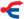本文介绍的是影族公猫。关于在中文版同名的河族母猫，请参见“暗星”。

Blackstar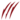泼皮猫 (Q649)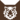虎族 (Q645)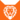狮族 (Q646)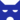影族 武士影族 副族长
（继任自断星 (Q2583)影族 武士影族 副族长
（继任自煤毛 (Q2731)影族 族长
（继任自虎星 (Q712)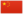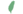小黑非官 Blackkit  小黑非官
 黑爪 Blackpaw  黑掌
 黑脚 Blackfoot  黑足
 黑星 Blackstar  黑星

Q2658：影族公猫，自《呼唤野性》登场的角色

## 细节

### 外貌

• 他是一只高:34而巨大:44、琥珀色眼睛的白色公猫，:封面有着乌黑色的脚掌，其中一只上有六个趾头。他有着光滑的皮毛:42和宽阔的胸膛；:36他的耳朵外缘为黑，但耳背为白。:封面
• 他生前有着伤痕累累:44的残破皮毛。:259
• 凯特认为他的眼睛是琥珀色的。

### 趣闻

• 他可能属于一个多趾猫世家，他的其中一只黑色脚爪上有六个脚趾。
• 他是第一只两度担任副族长的猫，分别辅佐断星:141和虎星。:6
• 他成为副族长时并没有指导过学徒。:257
• 他是少数放弃族长名并变更回武士名的猫之一，:84虽然之后他又决定恢复信仰并改回族长名。:127
• 由于他和雾星之间存在弑之仇，后者拒绝与他的族群结盟。:4章

## 登场书目

• 呼唤野性 (Q57)[配角][首次登场🐱]
• 寒冰烈火 (Q59)[配角]
• 疑云重重 (Q60)[配角]
• 风起云涌 (Q65)[对话提及]
• 险路惊魂 (Q66)[配角]
• 力挽狂澜 (Q67)[配角]
• 午夜追踪 (Q139)[配角]
• 新月危机 (Q140)[配角]
• 重现家园 (Q141)[配角]
• 星光指路 (Q142)[配角]
• 黄昏战争 (Q143)[配角]
• 日落和平 (Q144)[配角]
• 预视力量 (Q152)[配角]
• 暗河汹涌 (Q153)[配角]
• 驱逐之战 (Q154)[配角]
• 天蚀遮月 (Q155)[配角]
• 暗夜长影 (Q156)[配角]
• 拂晓之光 (Q157)[配角]
• 第四学徒 (Q158)[配角]
• 战声渐近 (Q159)[配角]
• 暗夜密语 (Q160)[配角]
• 月光印记 (Q161)[配角]
• 武士归来 (Q162)[配角]
• 群星之战 (Q163)[配角]
• 学徒探索 (Q170)[配角]
• 烈焰焚河 (Q174)[配角]
• 风暴来袭 (Q175)[配角]
• 火星的探索 (Q176)[配角]
• 黄牙的秘密 (Q180)[配角]
• 高星的复仇 (Q181)[配角]
• 黑莓星的风暴 (Q182)[配角][死亡时刻✝]
• 虎心的阴影 (Q185)[对话提及]
• 鸦羽的拷问 (Q186)[配角]
• 灰条的誓言 (Q4057)[配角]
• 豹星的荣光 (Q4426)[配角]
• 一星的告解 (Q4826)[配角]
• 冬青叶的故事 (Q187)[猫物闲角][对话提及]
• 雾星的征兆 (Q188)[对话提及]
• 虎掌的愤怒 (Q190)[配角]
• 叶池的祈愿 (Q191)[猫物闲角]
• 鸽翅的沉默 (Q192)[配角]
• 蛾翅的秘密 (Q3628)[配角]
• 黑脚的审判 (Q4336)[主人公][主要角色][视角人物]
• 河族阴云 (Q4311)[配角]
• 变革之风 (Q4411)[配角]
• 逐出影族 (Q4769)[配角]
• 武士回归 (Q209)[配角]
• 进入森林 (Q210)[配角]
• 族群的秘密 (Q199)[追溯修订]
• 族群的猫 (Q200)[配角]
• 族群的战争 (Q202)[配角]
• 终极指南 (Q204)[视角人物]
• 族群决定 (Q4580)[配角]
• 超越守则：光灵的恻隐之心 (Q4321)[配角]
• ## 周边

更多资料：黑星/周边
【系列2】黑星和带棍松鸦羽
• 系列2——炭毛亮心、黑星和带棍松鸦羽乌爪沙风
• 尽管在许多方面都非常不同，这些公猫都在他们的族群迫切需要时提供了服务。尽管黑星听从了影族最为残忍的族长们的命令，但在他上台后，他恢复了影族的荣耀和高尚。而当松鸦羽得知预言承诺了比星族更强大的力量时，他在一根古老棍子的帮助下，开始从黑暗中拯救族群。
• 长约7厘米。
• 一组约12.99美金；整套三组约38.97美金。

## 脚注

1. 因放逐而离开
2. 曙云和高罂都是他的学徒，但存在时间轴上的矛盾。高罂未登场于《黄牙的秘密》，但在《寒冰烈火》中已是武士；在《呼唤野性》，曙云是猫后，但和她一起成为学徒的小云仍是学徒。由于时间轴如此混乱，因此很难判定到底谁是黑星的第一个学徒。

## 参考文献

这篇文章基于CC BY-SA 3.0许可使用了猫武士维基（英语）Blackstar一文中的部分内容。